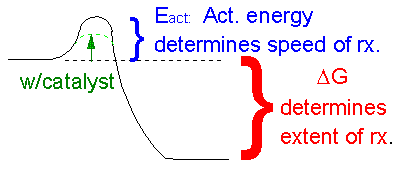# E. Summary

$$\newcommand{\vecs}{\overset { \rightharpoonup} {\mathbf{#1}} }$$ $$\newcommand{\vecd}{\overset{-\!-\!\rightharpoonup}{\vphantom{a}\smash {#1}}}$$$$\newcommand{\id}{\mathrm{id}}$$ $$\newcommand{\Span}{\mathrm{span}}$$ $$\newcommand{\kernel}{\mathrm{null}\,}$$ $$\newcommand{\range}{\mathrm{range}\,}$$ $$\newcommand{\RealPart}{\mathrm{Re}}$$ $$\newcommand{\ImaginaryPart}{\mathrm{Im}}$$ $$\newcommand{\Argument}{\mathrm{Arg}}$$ $$\newcommand{\norm}{\| #1 \|}$$ $$\newcommand{\inner}{\langle #1, #2 \rangle}$$ $$\newcommand{\Span}{\mathrm{span}}$$ $$\newcommand{\id}{\mathrm{id}}$$ $$\newcommand{\Span}{\mathrm{span}}$$ $$\newcommand{\kernel}{\mathrm{null}\,}$$ $$\newcommand{\range}{\mathrm{range}\,}$$ $$\newcommand{\RealPart}{\mathrm{Re}}$$ $$\newcommand{\ImaginaryPart}{\mathrm{Im}}$$ $$\newcommand{\Argument}{\mathrm{Arg}}$$ $$\newcommand{\norm}{\| #1 \|}$$ $$\newcommand{\inner}{\langle #1, #2 \rangle}$$ $$\newcommand{\Span}{\mathrm{span}}$$

Whether reactions are spontaneous in a given direction depends not just if the reaction is exothermic (since some reactions are endothermic) or just entropy (since some reactions that increase the number of microstates or energy dispersal occur spontaneously) but on some combination of both, as illustrated in the table below.

 reaction Δenthalpy (ΔH) Δentropy (ΔS) favored/disfavored combustion of fuels exothermic (<0) positive (>0) always favored various exothermic (<0) negative(<0) depends various endothermic (>0) positive (>0) depends synthesis of sugars from CO2 and H2O endothermic (>0) negative (<0) always disfavored

Once again, the total ΔG is a function of two thermodynamic parameters, ΔH and ΔS. You will remember the following equations from general chemistry:

ΔG = ΔH - TΔS and ΔGo = ΔHo - TΔSo

which describes the free energy, enthalpy, and entropy for any condition (ΔG) and for the standard state (ΔGo). Previously, we saw that ΔG was a function of two other terms that were more directly related to the actual chemistry of the system - the equilibrium constant, Keq, and the concentrations of reactants and products.

ΔG = ΔGo + RTlnQ = ΔGo + RTln ([P][Q])/([A][B])

Summary: ΔG determines if a reaction goes spontaneously in any given direction. If ΔG < 0, the reaction goes from reactants to products - to a state when products have a lower energy than reactants - like a ball rolling down a hill. Many factors contribute to ΔG, including RTln Q, ΔGo and ΔH. These are all energy terms and hence they will favor product formation if they are < 0. The other term which affects ΔG is ΔS. Entropy favors a product if ΔS >0. ΔS is not an energy term, but notice in the above equation that the term containing the contribution from entropy is -TΔS , where T is the temperature. This term is < 0, and favors product, if ΔS is positive (>0).

One thing that we haven't studied is the speed (not extent) of a reaction. They are two very different concepts. Consider for example the simple reaction of octane with oxygyen.

2C8H18(l) + 25O2(g) --> 16CO2(g) + 18H2O(g).

This reaction is clearly exothermic (ΔH < 0) and proceeds with an increase in disorder (ΔS>0). Therefore, it is very favored in the forward direction. But you all know from experience that if you pour gasoline on to a table in the presence of air, nothing seems to happen. How can this be if the reaction is so favorable that it is essentially irreversible? A look at the diagram below shows how we can rationalize this behavior. If you think about it , the first thing that must happen in the reaction above is that some bonds in the products must be broken. This is clearly an endothermic process. Energy (through collisions) must be put into the reactants to do this. The molecules then go to a higher energy state. Eventually a few molecule then start forming stable bonds found in the product and in doing so, they release a lots of energy, in the form of heat. This released heat increases the temperature of the container, causing the molecules to have increased kinetic energy, increasing the number of collisions between reactant molecules which cause a cascade of bond breaking and making, resulting in a huge release of energy. This is why a spark is needed to "get the reaction going", even though it is greatly favored.ANIMATION: ACTIVATION ENERGY AND A BALL ON A HILL